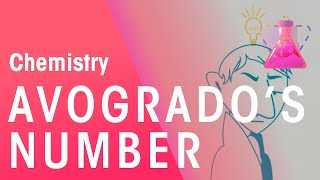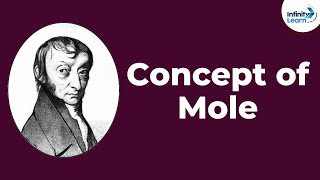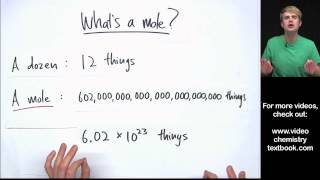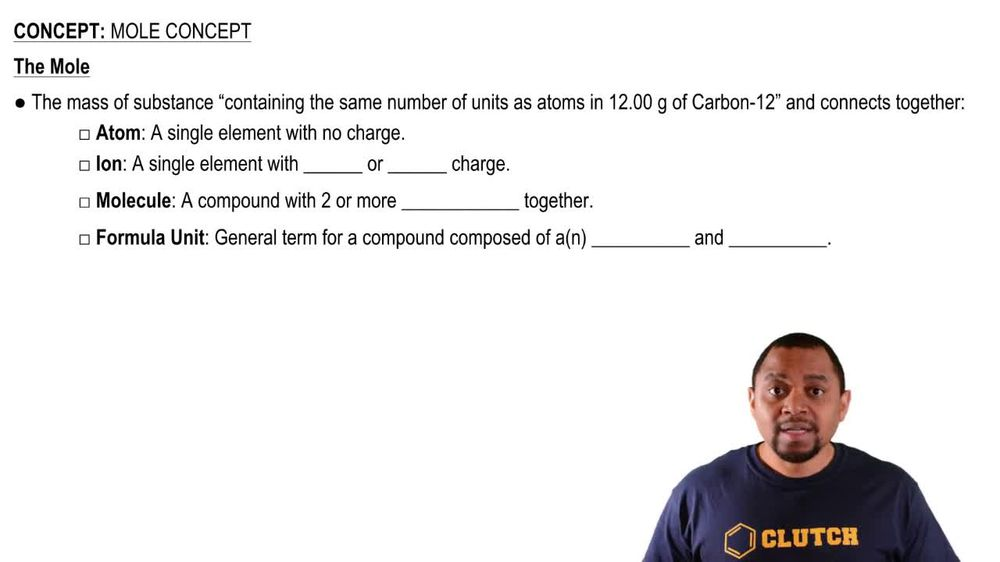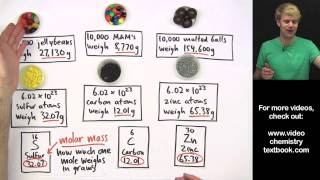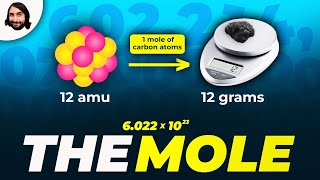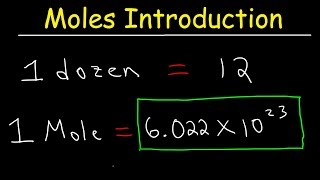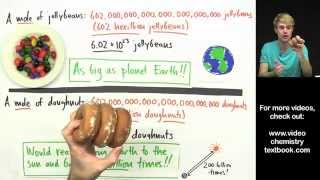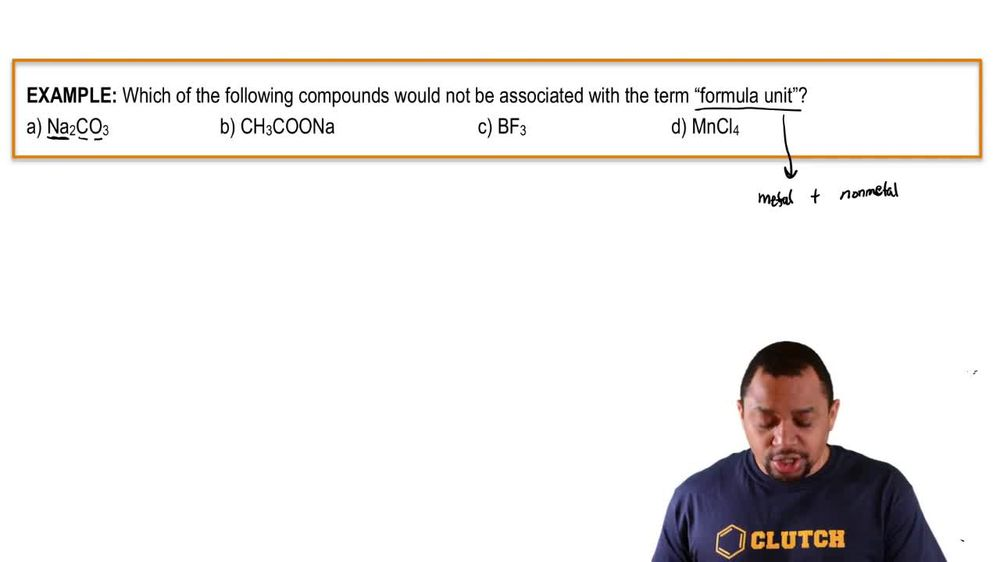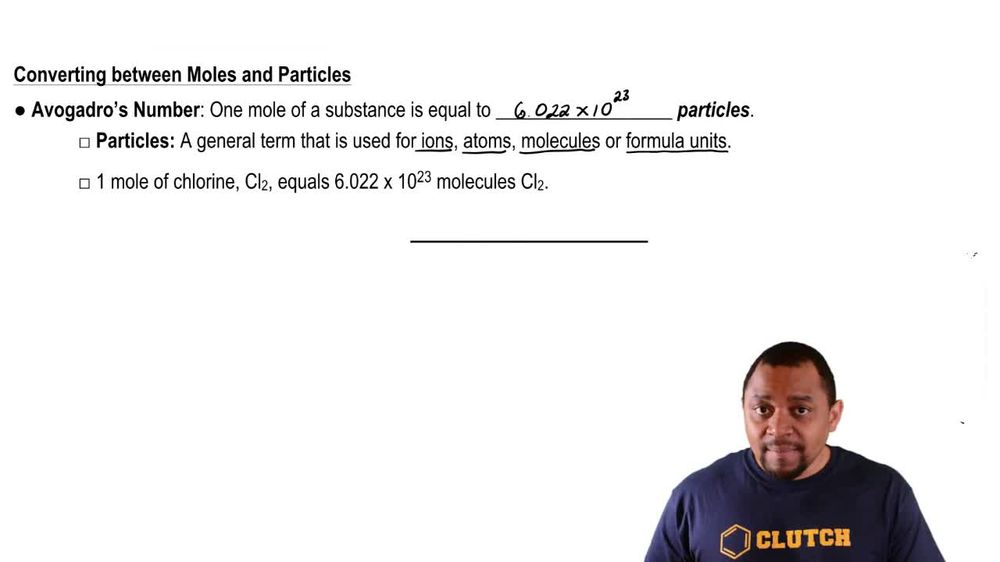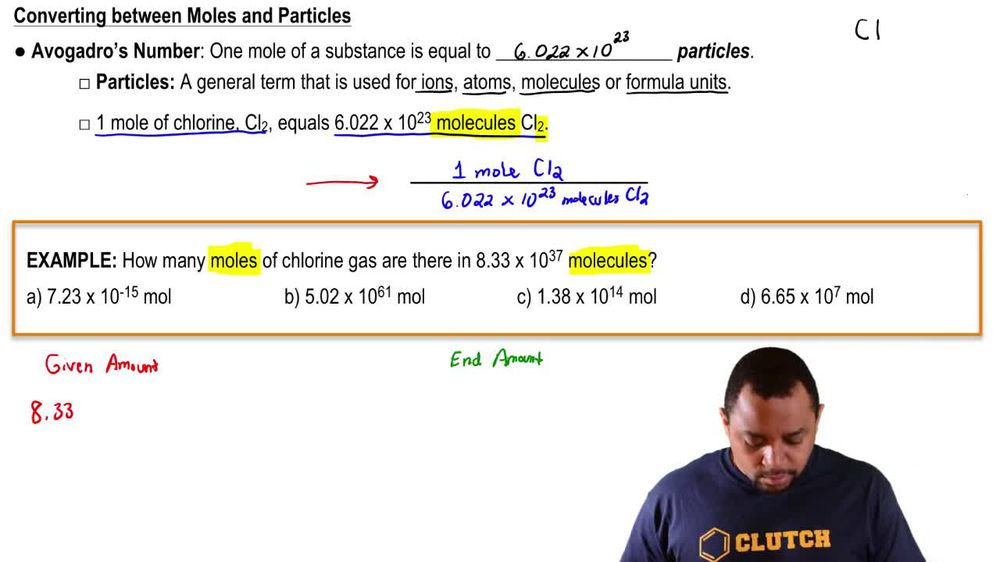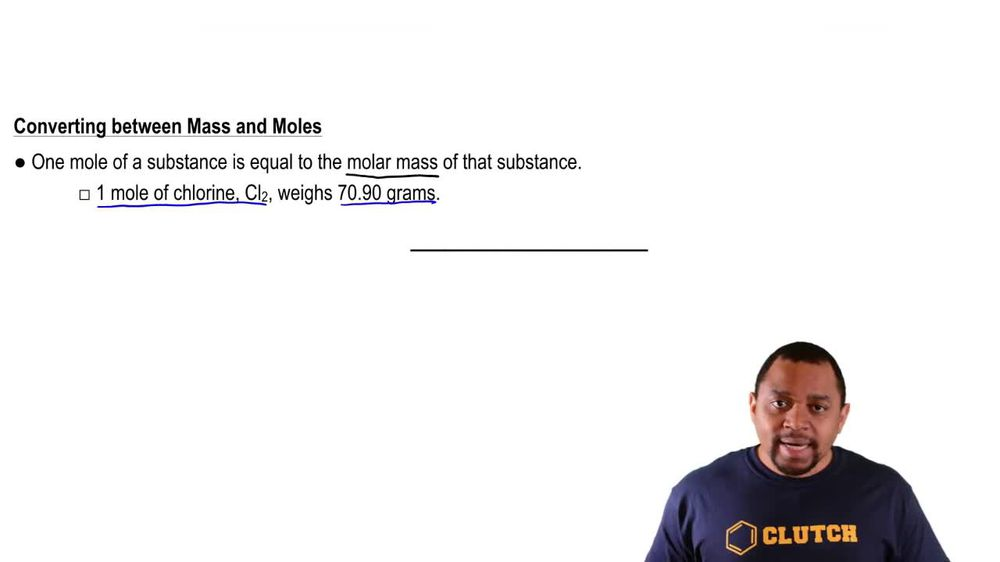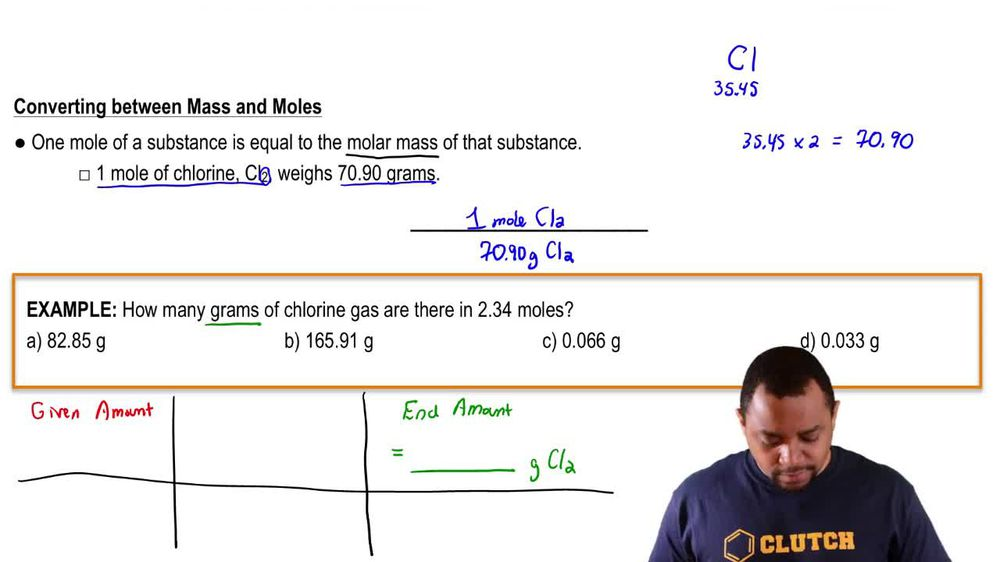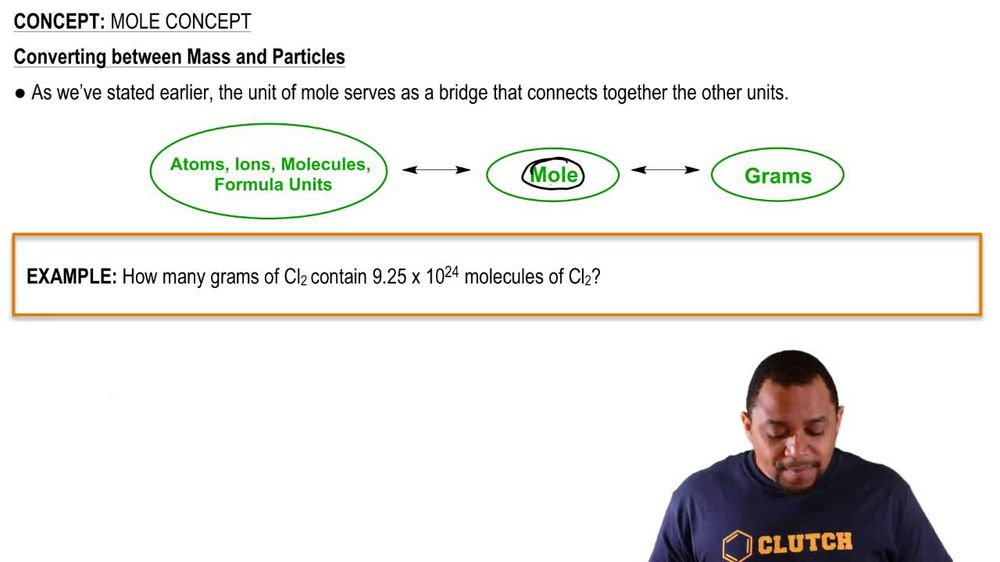Start typing, then use the up and down arrows to select an option from the list.
1. 2. Atoms & Elements2. Mole Concept# Mole Concept Example 1

by Jules Bruno
117 views
0
So in this example question, it says which of the following compounds would not be associated with the term formula unit. So remember, Formula Unit is just a general term we're going to use in terms of a compound that consists, possesses ah, metal plus nonmetal. So we just have to look for the choice that does not contain a medal with nonmetal. If you look at Option A, we have N a C and O. N A is a medalist, sodium carbon and oxygen are both non metals, so this would represent a compound that could be termed a formula unit. Next, we have this long compound here, but again it has sodium in it, and all these elements here are non metals. So this could also use the term formula unit. See, though, see has B, which is boron. Boron is a metal Lloyd, and it has F, which is flooring, which is a non metal. So this would be our answer we could not call. We cannot associate the term formula unit with this compound because it contains a metal Lloyd and not a metal. And then finally we have m n and C. L M n is mag unease, which is a transition metal, and see Ellis chlorine, which is a non metal, so this could be associated with the term formula unit. So, out of all the choices on Lee, Option C couldn't be associated with the term Formula unit.